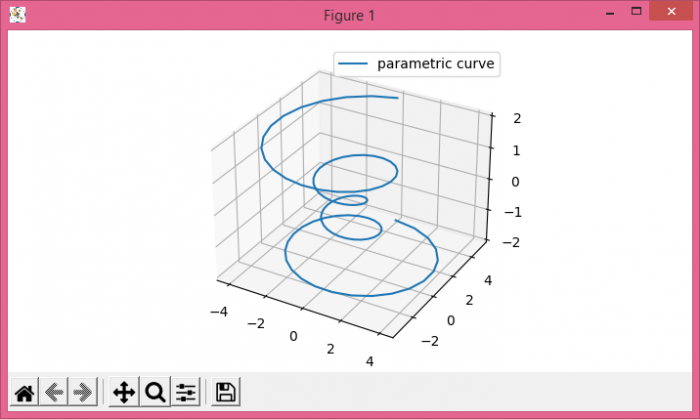# How can I make a simple 3D line with Matplotlib?

To make a simple 3D line with matplotlib, we can take the following steps −

• Create a new figure or activate an existing figure.

• Add axes to the figure as part of a subplot arrangement.

• Create data points for theta, z, r, x and using numpy.

• Plot x, y and z using plot() method.

• Place a legend on the figure using legend() method.

• To display the figure, use show() method.

## Example

import numpy as np
from matplotlib import pyplot as plt
plt.rcParams["figure.figsize"] = [7.00, 3.50]
plt.rcParams["figure.autolayout"] = True
fig = plt.figure()
theta = np.linspace(-4 * np.pi, 4 * np.pi, 100)
z = np.linspace(-2, 2, 100)
r = z**2 + 1
x = r * np.sin(theta)
y = r * np.cos(theta)
ax.plot(x, y, z, label="parametric curve")
ax.legend()
plt.show()

## Output